# Physical basis of limit calculations

There are two principal methods of estimating upper bounds on tropical cyclone intensity, but they are closely related. The first method was pioneered by Miller (1958) and involves first estimating the maximum temperature that can be achieved in the eyewall given the thermodynamic properties of air near the sea surface, and then estimating the maximum temperature that can be achieved in the eye by compressional warming of sinking air. Miller's technique has been expanded upon and improved by Holland (1996). The second technique uses the energy cycle of the storm to estimate the maximum possible surface wind speed and was developed by Emanuel (1986, 1995). The central pressure can also be estimated by assuming a particular radial profile of azimuthal wind inside the eye, but this is not necessary if all that is desired is the maximum wind speed. We will describe the energy method here, as it is simpler to describe, but we emphasize that the two techniques yield similar results.

The energy cycle is illustrated in Figure 1. Air spirals in toward the storm center and, owing to the large surface wind speeds, rapidly acquires heat from the underlying ocean. This additional heat shows up mostly as an increase in the humidity, rather than the temperature, of the air. (When water evaporates from the ocean, it takes heat out of the ocean and this energy then resides in the water vapor content of the air. Because it does not immediately increase the temperature of the air, it is called latent heat. Ultimately, when the water vapor condenses inside clouds, the latent heat is converted to sensible heat and the temperature then actually increases somewhere in the system.) The total increase in heat content on this leg is proportional to the increase in water vapor content and the decrease in pressure; this latter effect is called heat input by isothermal expansion.''

Once the air reaches the eyewall, it turns upward and quickly rises through the eyewall to around 15-18 km altitude, where it then flows outward. The ascent is nearly adiabatic; that is, now net heat is added in this leg, though there are large conversions of latent to sensible heat. The heat is exported to the storm's environment and ultimately lost by radiation to space.

A famous theorem of thermodynamics states that only a fraction of the heat put into the heat engine is available for generation of wind energy. This fraction is called the thermodynamic efficiency,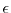, and is defined by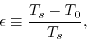(1)

where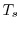is the temperature of the heat source (in this case, the ocean surface) and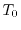is the average temperature at which heat is exported from the system. (Both temperatures are measured as absolute, or Kelvin temperature.) The taller a hurricane is, the lower the temperature at its top and thus, from (1), the great the thermodynamic efficiency. Moreover, if we know how much heat air has in the eyewall and we know the vertical temperature structure of the air in the environment of the storm, then we can calculate how high air will rise in the eyewall and thus we can calculate. This, together with sea surface temperature, gives us, from (1). In a typical hurricane,is about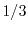.

The rate of input of available energy, into the hurricane from the sea surface, for each square meter of sea surface covered by the storm, is given by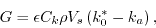(2)

where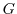stands for generation,''is a dimensionless coefficient called the enthalpy transfer coefficient,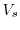is the surface wind speed, andand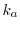are the enthalpies of the ocean surface and the atmosphere near the surface, respectively. To get the entire energy input, it is necessary to add up the contributions given by (2) over each square meter of the ocean surface affected by the storm, but here we are going to assume that this sum is dominated by the contribution near the radius of maximum wind, whereis large.

What happens to all this energy that is generated by heat transfer from the sea surface? When the storm has reached a nearly steady condition (on which its intensity is no longer changing), almost all of the energy generated is used up by friction acting between the powerful winds and the sea surface. The rate of mechanical dissipation in the system, for each square meter of ocean surface, is given by(3)

where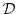stands for dissipation'' and the other symbols are the same as in (2), butis a different coefficient, called the drag coefficient. Once again, it is necessary to sum (31) over each square meter of ocean surface affected by the storm, but we will again assume that it is dominated by the contributions near the radius of maximum wind.

Equating generation, given by (2), to dissipation, given by (3), gives an expression for the wind speed: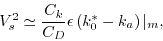(4)

where the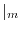reminds us that we shall evaluate the quantities near the radius of maximum wind.

It is important to note here that (4) can be derived much more rigorously than has been done here and that when this is done, it turns out thatgiven by (4) is indeed an expression for the maximum surface wind speed (see Emanuel, 1995). It is also important to recognize that the derivation of (4) depends in no way on what happens inside the eye of the storm.

To actually evaluate (4), it is necessary to know several things. First, we have to know the ratio of heat to momentum exchange coefficients,. Unfortunately, no measurements of either of these coefficients have been made at hurricane wind speeds. For now, it is assumed that this ratio is 1. Another thing we have to know is the saturation enthalpy of the ocean surface,. This depends mostly on sea surface temperature, but also on sea level pressure at the radius of maximum winds. To get this, it is necessary to assume something about the distribution of wind outside the radius of maximum winds. The calculation of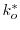is not terribly sensitive to what one assumes about this distribution, as long as it is reasonable. One also has to estimate the enthalpy of the air just above the sea surface,, at the radius of maximum winds. This must be at least as large as the entropy of unperturbed boundary layer air, and it depends on temperature, pressure, and relative humidity. Both Holland (1996) and Emanuel (1995) assume that the air temperature is closely linked to the sea surface temperature. Holland assumes that the relative humidity is 85%, while Emanuel assumes that it is the same as that of the unperturbed environment (usually around 80%). Finally, one has to estimate. To do this, one has to first estimate, the temperature at which air flows out of the top of the storm; this is also equivalent to estimating the altitude of the storm top. This accomplished by finding out how high up in the eyewall the air remains warmer than the distant environment, and is straightforward to assess given the vertical profile of temperature in the storm environment.

Storm intensity is also often measured by its central pressure. One method of getting the central pressure is to first assume that the eye is in solid body rotation,'' i.e., that the swirling wind increases linearly with radius out to the radius of maximum wind. Taking the wind field to be balanced by the radial pressure gradient is an excellent approximation in the hurricane eye, and so one can easily calculate the central pressure given the pressure at the radius of maximum winds and the maximum wind speed, both of which we know from the aforementioned reasoning. This gives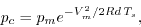(5)

where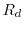is the gas constant for air.

It should be noted that there is no universal agreement that this is the best way to estimate central pressure, and Holland (1996), among others, offers a very different technique.

To get an idea of how the storm intensity depends on sea surface and storm outflow temperature, examine Figure 2, which uses (4) and (5) to calculateand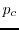given ocean temperature and, and assuming that the relative humidity under the eyewall is 75%. Both measures show intensity increasing with sea surface temperature, and the rate of increase also gets steeper at higher sea surface temperature. Note also that at very high sea surface temperature and low outflow temperature, there are no solutions to the equations. This is the hypercane'' regime. Numerical simulations in this regime produce very intense storms (with wind speeds near the speed of sound), and it has been speculated that such storms may have formed over pools of very hot water created by large asteroid impacts with the ocean (Emanuel et al., 1995). But we do not have to worry about hypercanes otherwise!

One prediction of the form of limiting theory presented here is that the intensity of tropical cyclones should depend on the ratio of surface exchange coefficients,(see eqn. 4). As mentioned above, we do not know very well what this is in nature, but we are free to specify it in computer models of hurricanes. Figure 3 shows the results of running two quite different computer models and comparing the results with the theory (see Emanuel, 1995, for details). As predicted, the storm intensity does increase with.

Kerry Emanuel 2011-11-22